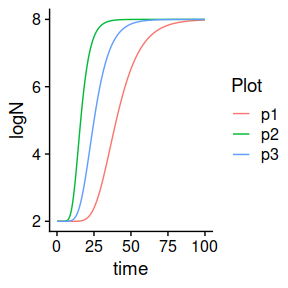# Introduction

The biogrowth package for R allows for modelling of microbial growth using predictive microbiology. This vignette is an addition to the main vignette of the package, giving further detail on how tu use the functions in biogrowth to prepare publication ready figures. Here, we assume the reader is already familiar with biogrowth.

library(biogrowth)
library(tidyverse)
#> ── Attaching packages ─────────────────────────────────────── tidyverse 1.3.0 ──
#> ✓ ggplot2 3.3.2     ✓ purrr   0.3.4
#> ✓ tibble  3.0.4     ✓ dplyr   1.0.2
#> ✓ tidyr   1.1.2     ✓ stringr 1.4.0
#> ✓ readr   1.4.0     ✓ forcats 0.5.0
#> ── Conflicts ────────────────────────────────────────── tidyverse_conflicts() ──
#> x dplyr::lag()    masks stats::lag()

Several functions in the package make use of stochastic algorithms. For reproducibility, we will set the seed of R’s internal pseodu-random number generator (PRNG) to some arbitrary value

set.seed(1241)

Biogrowth uses the cowplot package and the cowplot theme as default. Therefore, the vanilla figures produced by biogrowth already have a nice clean theme and could be used in a publication as is. However, more control over formatting options can be needed in order to standardize plots or when preparing figures for a specific journal.

Cowplot is a package that works with gplot2, and therefore the plots can be manipulated in the same way. Arguments that control the way that data is represented by geoms such as:

• colour
• size
• linetype

would normally go inside the ggplot call. In biogrowth, a modified version of these arguments can be set by providing additional arguments to plotting methods in biogrowth.

# Specify the model
my_model <- "modGompertz"

# Define the starting parameters (mu, lambda and C), and the initial count in a list
my_pars <- list(logN0 = 2, C = 6, mu = .2, lambda = 25)

# Define the storage times
my_time <- seq(0, 100, length = 1000)

# Call to predict_isothermal_growth using the arguments defined above
static_prediction <- predict_isothermal_growth(my_model, my_time, my_pars)

# Plotting
plot(static_prediction)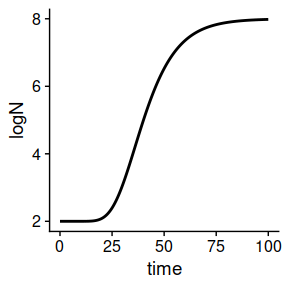By setting the optional arguments line_size, line_col, and line_size to plot(), we can control the size, colour, and linetype of the plot.

# Plotting
plot(static_prediction,
line_col = "red", # also takes strings or hexadecimal code, try entering "#56B4E9" for blue
line_size = 1,
line_type  = "dashed")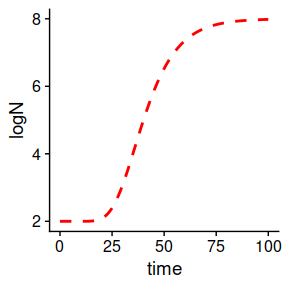## Dynamic growth plotting

The same attributes can be set via the plot() function for the primary axis of dynamic_prediction()

# Example data from the main vignette
my_conditions <- tibble(time = c(0, 5, 40),
temperature = c(20, 30, 35),
pH = c(7, 6.5, 5)
)
my_primary <- list(mu_opt = 2,
Nmax = 1e8,
N0 = 1e0,
Q0 = 1e-3)

sec_temperature <- list(model = "Zwietering",
xmin = 25,
xopt = 35,
n = 1)

sec_pH = list(model = "CPM",
xmin = 5.5,
xopt = 6.5,
xmax = 7.5,
n = 2)

my_secondary <- list(
temperature = sec_temperature,
pH = sec_pH
)

my_times <- seq(0, 50, length = 1000)

# Calling the function
dynamic_prediction <- predict_dynamic_growth(my_times,
my_conditions, my_primary,
my_secondary)

The line of the predicted values can be modified via additional arguments to plot()

plot(dynamic_prediction,
line_col = "blue")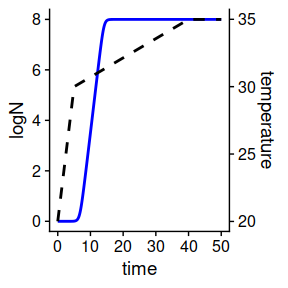## Plotting of fits

To show the additional options that can be supplied to fit_isothermal_growth(), and fit_dynamic_growth() we use the example from the main vignette

# Example data from the main vignette
my_data <- tibble(time = c(0, 25, 50, 75, 100),
logN = c(2, 2.5, 7, 8, 8))
my_model <- "Baranyi"
known <- c()
start = c(logNmax = 8, lambda = 25, logN0 = 2, mu = .2)

# Fitting
static_fit <- fit_isothermal_growth(my_data, my_model,
start, known
)

and plot this with additional arguments for the color of the points, and a different line_type

# Plotting
plot(static_fit,
point_col = "lightgreen",
point_size = 3,
line_type = 3)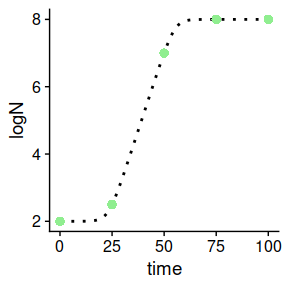This example uses the tri-linear growth model to make a stochastic prediction with the predict_stochastic_growth() function. Note that the same arguments can be set for fit_MCMC_growth()

# Example data from the main vignette

my_model <- "Baranyi"
my_times <- seq(0, 30, length = 100)
n_sims <- 100  # Just as an example, should be increased in actual analyses

pars <- tribble(
~par, ~mean, ~sd, ~scale,
"logN0", 0, .2, "original",
"mu", 2, .3, "sqrt",
"lambda", 4, .4, "sqrt",
"logNmax", 6, .5, "original"
)

stoc_growth <- predict_stochastic_growth(my_model, my_times, n_sims, pars)

We can supply plot() with the arguments that control the size, type and colour of the q50 line, and in addition:

• ribbon80_fill: the colour of the ribbon denoting the space between the 10th and 90th quantile
• ribbon90_fill: the colour of the ribbon denoting the space between the 5th and 95th quantile
• alpha80: the opacity of the ribbon80_fill
• alpha90: the opacity of the ribbon90_fill
plot(stoc_growth,
line_type = 3,
ribbon80_fill = "#00dbdb",
ribbon90_fill = "#00dbdb",
alpha80 = .5,
alpha90 = .4)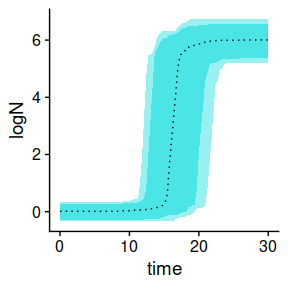Note that ribbon80_fill and ribbon90_fill can be set to different colours, but this is not always an improvement

plot(stoc_growth,
line_type = 3,
ribbon80_fill = "red",
ribbon90_fill = "grey",
alpha80 = .5,
alpha90 = .4)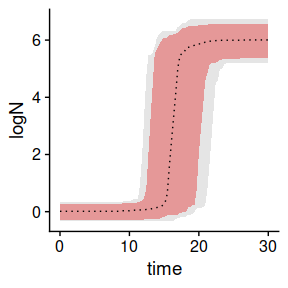## Distributions of times to reach a certain count

#COMMENT: Gives warning:valuescollapsing to unique ‘x’ or sometimes other error: valuesError in approx(.$$logN, .$$time, log_count) : #COMMENT need at least two non-NA values to interpolate

We use the result of predict_stochastic_growth() stored in stoc_growth from the example above.

time_distrib <- distribution_to_logcount(stoc_growth, 4)

The prediction of times to reach a specified count is shown in a histogram. We can adjust the binwidth of the histogram by setting the bin_width argument. In this example values between 1 and 3 would be representative.

plot(time_distrib, bin_width = 2)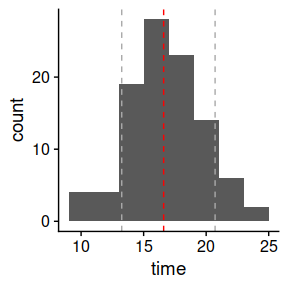## Combining plots into subplots

The function plot_grid() of cowplot can be used to combine different plots into a grid.


library(cowplot)

p1 <- plot(static_fit,
point_col = "lightgreen",
point_size = 3,
line_type = 3)

p2 <- plot(dynamic_prediction,
line_col = "blue")

p3 <- plot(stoc_growth,
line_type = 3,
ribbon80_fill = "#00dbdb",
ribbon90_fill = "#00dbdb",
alpha80 = .5,
alpha90 = .4)

p4 <- plot(stoc_growth,
line_type = 3,
ribbon80_fill = "red",
ribbon90_fill = "grey",
alpha80 = .5,
alpha90 = .4)

# Making a nice grid of plots
plot_grid(p1, p2, p3, p4, labels = "AUTO")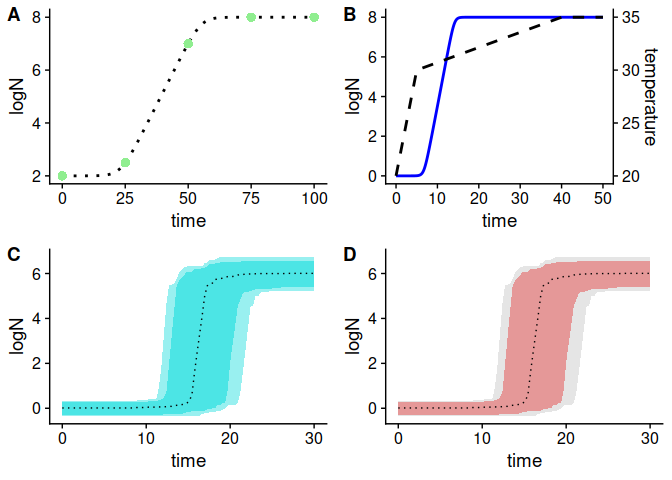# Additional changes to the plotting area

Properties of the plotting area are controlled by ggplot2 via additional layers. We can do the same here, with additional layers to the plot() function. For instance, to change the thickness of the x- and y-axis we use a theme layer adressing axis.line to change its colour and size.

# Axis line thickness via theme()
plot(static_prediction, line_col = "red") +
theme(axis.line = element_line(colour = 'grey', size = 1)) + # Adjust size and colour to taste
xlab("Time")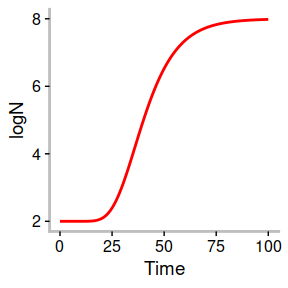In some cases, the automatic scaling that ggplot2 uses might not be optimal. We can use the coord_cartesian() function to change the limits of the x- and y-axis

# From the previous example
plot(static_prediction, line_col = "red") +
theme(axis.line = element_line(colour = 'grey', size = 0.8)) + # Adjust size and colour to taste
coord_cartesian(xlim = c(0, 100), ylim = c(0, 10)) # changing the axis limits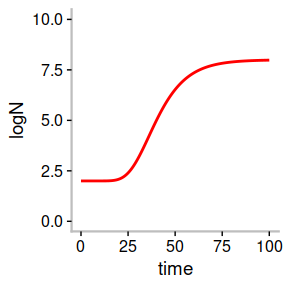## Saving and reshaping plots

ggsave() will automatically save the last plot to a specified location. It needs a filename as a string, for instance “static_prediction.pdf” to save the figure as a pdf. It also needs a location to save to (defaults to the working directory), and optionally the user can set dimentions and units.

# We save the plot as p1, notice that it does not get drawn now
p1 <- plot(static_prediction, line_col = "red") +
theme(axis.line = element_line(colour = 'grey', size = 0.8))

# We save P1 as .pdf, as a 20x 20 cm square
# ggsave("static_prediction.pdf", p1, width = 20, height = 20, units = "cm")

# Full manual control

By accessing the data directly, the experienced user can get full control over plotting:

# Vanilla biogrowth figure
static_prediction$simulation %>% ggplot(., aes(x= time, y =logN )) + geom_line() + theme_cowplot()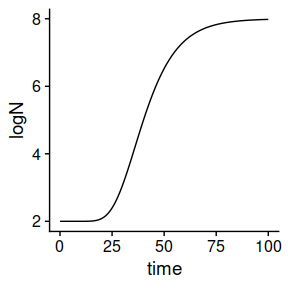# Directly accessing the simulation data from static_prediction P <- ggplot(static_prediction$simulation, aes(x= .data$time, y =.data$logN)) +
geom_line(color="red") +
theme_cowplot()

P +
coord_cartesian(xlim = c(0, 100), ylim = c(0, 10)) +
theme(axis.line = element_line(colour = 'black', size = 0.4))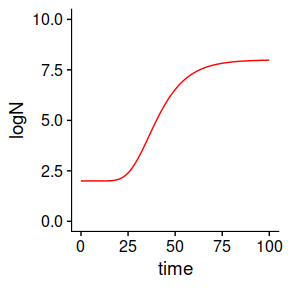# Plotting
ggplot(stoc_growth$quantiles, aes(x = .data$time)) +
geom_ribbon(aes(ymin = .data$q10, ymax = .data$q90), fill = "red",  alpha = .3) +
geom_ribbon(aes(ymin = .data$q05, ymax = .data$q95), fill = "red",  alpha = .2) +
geom_line(aes(y = .data$q50)) + xlab("Time") + ylab("logN") + cowplot::theme_cowplot()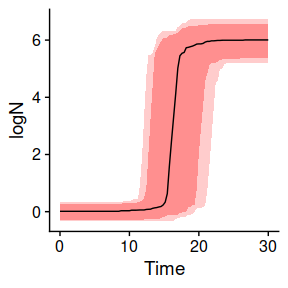## Comparing predictions In order to compare the outcome of simulations with different parameters we can combine simulations into a single plot using the plot_grid() function from cowplot. # Plotting multiple plots together my_model <- "modGompertz" my_pars <- list(logN0 = 2, C = 6, mu = .2, lambda = 25) my_time <- seq(0, 100, length = 1000) static_prediction1 <- predict_isothermal_growth(my_model, my_time, my_pars) p1 <- plot(static_prediction1) my_pars <- list(logN0 = 2, C = 6, mu = .5, lambda = 10) my_time <- seq(0, 100, length = 1000) static_prediction2 <- predict_isothermal_growth(my_model, my_time, my_pars) p2 <- plot(static_prediction2) my_pars <- list(logN0 = 2, C = 6, mu = .3, lambda = 15) my_time <- seq(0, 100, length = 1000) static_prediction3 <- predict_isothermal_growth(my_model, my_time, my_pars) p3 <- plot(static_prediction3) # Plotting using the cowplot plot_grid function plot_grid(p1, p2, p3, labels = "auto")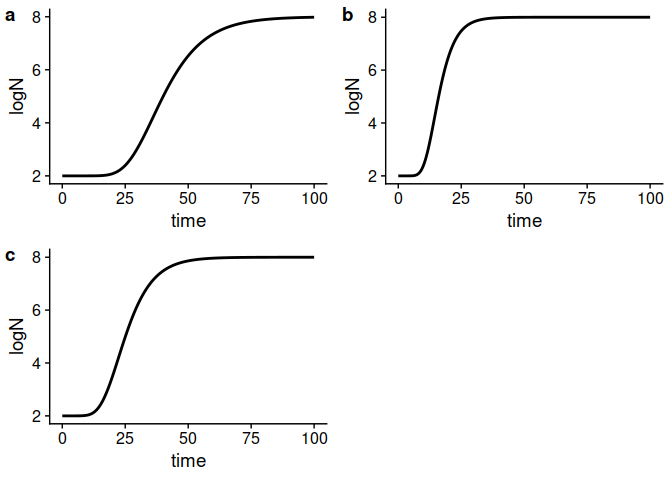Alternatively, one can combine the predicted counts into a single tibble and, then, plot all the growth curves in a single plot. bind_rows( tibble(Plot = rep("p1", 1000), time = static_prediction1$simulation$time, logN = static_prediction1$simulation$logN), tibble(Plot = rep("p2", 1000), time = static_prediction2$simulation$time, logN = static_prediction2$simulation$logN), tibble(Plot = rep("p3", 1000), time = static_prediction3$simulation$time, logN = static_prediction3$simulation$logN)) %>% ggplot(., aes(x = .data$time, y = .data$logN, col = .data$Plot)) +
geom_line() +
theme_cowplot()